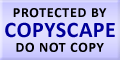Submit Assignment From Here
Name
:
Email
:
Phone
:
Country
:
Willing to pay US\$
:
Subject
:
Topic
:
Levels
:
:Hours
:

# Cost Volume Analysis Homework Help

Get online Cost Volume Analysis Homework Help by our experts at Assignment Hut. One of the important fields that we cover in Cost Management is the Cost Volume Analysis. It is one of the tools of cost management for taking short term decisions.

Cost volume analysis is one of the important components of cost management. It is a very simplified concept and helps in taking short term decisions in an organization. Cost Volume Analysis is an extension of Break-even theory and as it dwells on the concept of revenues and profits it is also known as Cost- Volume- Profit Analysis (CVP).

The breakeven theory states that at one point where the Total Revenues is equal to the Total Fixed and Variable costs at this point there is no profit and no loss.

We can also say that the Total profit is the difference between the Total Revenue and Total costs.

Total Profit = Total Revenue – Total costs (i.e. Fixed + variable costs)

### Important ingredients of the CVP are:

1. Selling price per unit
2. Variable cost per unit
3. Fixed costs
4. Volume or quantity of product

CVP analysis facilitates in calculating revenues where costs and quantum is given. It harps on maximizing profits after the breakeven point.

## Homework and Assignment Help on Cost Volume Analysis at Assignment Hut

Assignment Hut provides not only a helping hand in cost volume analysis in solving problems and assignments, but it also provides an in-depth knowledge of the concept and theories on which the concept is based.

So without further delay feel free to learn and understand concepts and theories with much ease and lesser time from certified experts!

 Submit Assignment From Here Accounting Homework Help

### Live Online Tutoring### Make Payment OnlineUS